# 2D Shearing in Computer Graphics | Definition | Examples

## 2D Transformations in Computer Graphics-

We have discussed-

• Transformation is a process of modifying and re-positioning the existing graphics.
• 2D Transformations take place in a two dimensional plane.

In computer graphics, various transformation techniques are-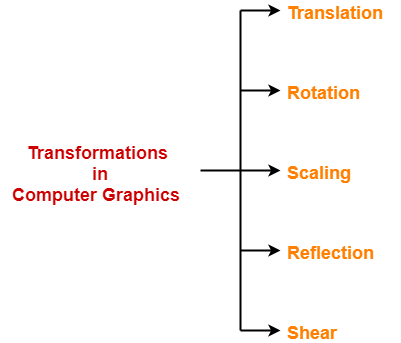## 2D Shearing in Computer Graphics-

 In Computer graphics, 2D Shearing is an ideal technique to change the shape of an existing object in a two dimensional plane.

In a two dimensional plane, the object size can be changed along X direction as well as Y direction.

So, there are two versions of shearing-1. Shearing in X direction
2. Shearing in Y direction

Consider a point object O has to be sheared in a 2D plane.

Let-

• Initial coordinates of the object O = (Xold, Yold)
• Shearing parameter towards X direction = Shx
• Shearing parameter towards Y direction = Shy
• New coordinates of the object O after shearing = (Xnew, Ynew)

### Shearing in X Axis-

Shearing in X axis is achieved by using the following shearing equations-

• Xnew = Xold + Shx x Yold
• Ynew = Yold

In Matrix form, the above shearing equations may be represented as-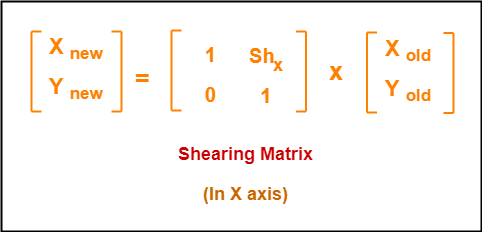For homogeneous coordinates, the above shearing matrix may be represented as a 3 x 3 matrix as-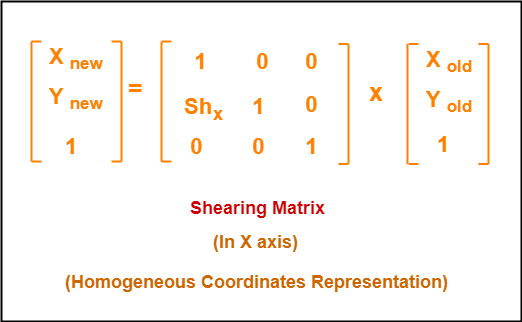### Shearing in Y Axis-

Shearing in Y axis is achieved by using the following shearing equations-

• Xnew = Xold
• Ynew = Yold + Shy x Xold

In Matrix form, the above shearing equations may be represented as-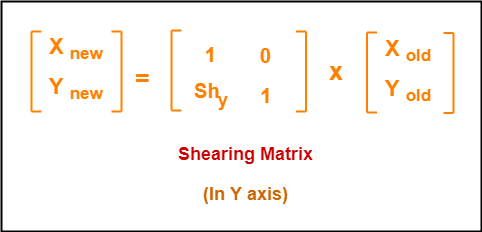For homogeneous coordinates, the above shearing matrix may be represented as a 3 x 3 matrix as-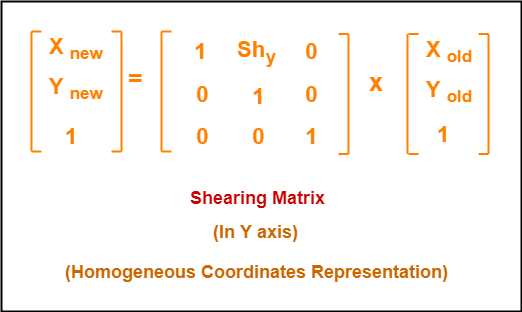## Problem-01:

Given a triangle with points (1, 1), (0, 0) and (1, 0). Apply shear parameter 2 on X axis and 2 on Y axis and find out the new coordinates of the object.

## Solution-

Given-

• Old corner coordinates of the triangle = A (1, 1), B(0, 0), C(1, 0)
• Shearing parameter towards X direction (Shx) = 2
• Shearing parameter towards Y direction (Shy) = 2

## Shearing in X Axis-

### For Coordinates A(1, 1)

Let the new coordinates of corner A after shearing = (Xnew, Ynew).

Applying the shearing equations, we have-

• Xnew = Xold + Shx x Yold = 1 + 2 x 1 = 3
• Ynew = Yold = 1

Thus, New coordinates of corner A after shearing = (3, 1).

### For Coordinates B(0, 0)

Let the new coordinates of corner B after shearing = (Xnew, Ynew).

Applying the shearing equations, we have-

• Xnew = Xold + Shx x Yold = 0 + 2 x 0 = 0
• Ynew = Yold = 0

Thus, New coordinates of corner B after shearing = (0, 0).

### For Coordinates C(1, 0)

Let the new coordinates of corner C after shearing = (Xnew, Ynew).

Applying the shearing equations, we have-

• Xnew = Xold + Shx x Yold = 1 + 2 x 0 = 1
• Ynew = Yold = 0

Thus, New coordinates of corner C after shearing = (1, 0).

Thus, New coordinates of the triangle after shearing in X axis = A (3, 1), B(0, 0), C(1, 0).

## Shearing in Y Axis-

### For Coordinates A(1, 1)

Let the new coordinates of corner A after shearing = (Xnew, Ynew).

Applying the shearing equations, we have-

• Xnew = Xold = 1
• Ynew = Yold + Shy x Xold = 1 + 2 x 1 = 3

Thus, New coordinates of corner A after shearing = (1, 3).

### For Coordinates B(0, 0)

Let the new coordinates of corner B after shearing = (Xnew, Ynew).

Applying the shearing equations, we have-

• Xnew = Xold = 0
• Ynew = Yold + Shy x Xold = 0 + 2 x 0 = 0

Thus, New coordinates of corner B after shearing = (0, 0).

### For Coordinates C(1, 0)

Let the new coordinates of corner C after shearing = (Xnew, Ynew).

Applying the shearing equations, we have-

• Xnew = Xold = 1
• Ynew = Yold + Shy x Xold = 0 + 2 x 1 = 2

Thus, New coordinates of corner C after shearing = (1, 2).

Thus, New coordinates of the triangle after shearing in Y axis = A (1, 3), B(0, 0), C(1, 2).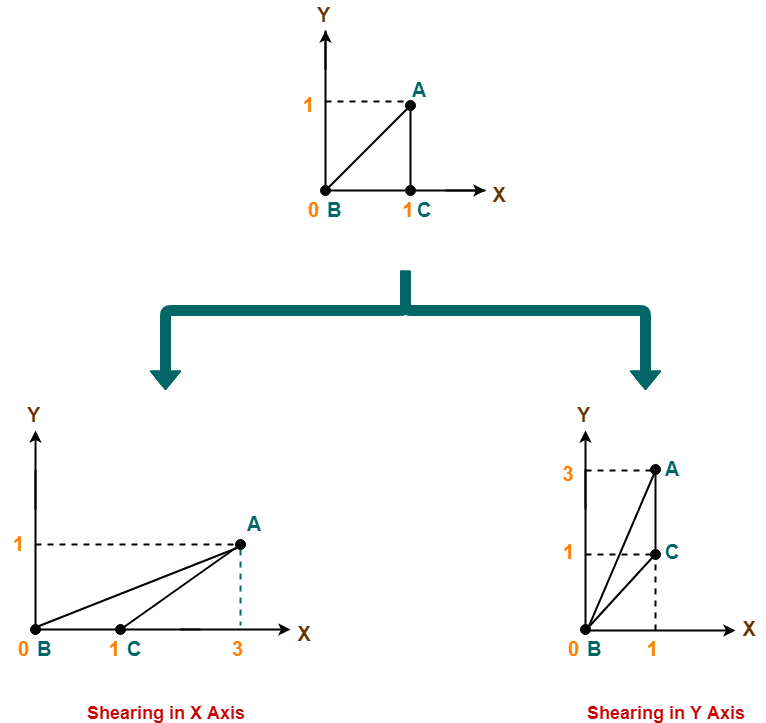To gain better understanding about 2D Shearing in Computer Graphics,

Watch this Video Lecture

Get more notes and other study material of Computer Graphics.

Watch video lectures by visiting our YouTube channel LearnVidFun.

SummaryArticle Name
2D Shearing in Computer Graphics | Definition | Examples
Description
2D Shearing in Computer Graphics is a process of modifying the shape of an object in 2D plane. Shearing Transformation in Computer Graphics Definition, Solved Examples and Problems.
Author
Publisher Name
Gate Vidyalay
Publisher Logo